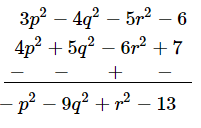# Subtract:

Question:

Subtract:
4p2 + 5q2 − 6r2 + 7 from 3p2 − 4q2 − 5r2 − 6

Solution:

Writing the terms of the given expressions (in the same order) in the form of rows with like terms below each other and subtracting column-wise: